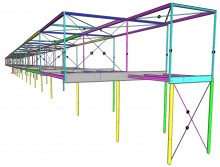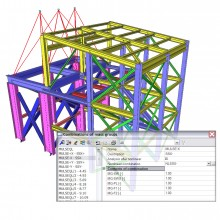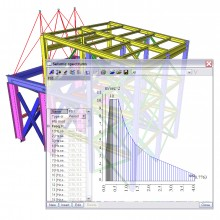# sens.22 - Sequential analysis

sens.22

## Highlights

 Non-linear combination added in a linear combination Linear stability calculation after a non-linear calculation Dynamic calculation after a non-linear calculation Eigenmode calculation with non-linearities Harmonic calculation with non-linearities Seismic calculation with non-linearitiesIn sequential analysis you can select a predefined sequence of different calculations, where the second one starts from the results of the first one. This means that the results from the first analysis are taken as the initial state for the second one.

The sequential analysis gives experienced users the possibility to obtain results that are not available through a single analysis. Two different types of sequential analysis are available.

The first type is a superposition of two different methods of calculation (e.g. linear + non-linear calculation). In other words, results of both calculations are added up. This is done for a non-linear combination which can be combined with a linear combination.

Second, the phased type is offered. This means that the second analysis starts where the first one ends; it takes the history of the structure into account. For this type, several couples of analyses are possible:

• Linear stability calculation after a non-linear calculation
•  Dynamic calculation after a non-linear calculation:
• An eigenmode calculation which takes non-linearities into account
• A harmonic calculation which takes non-linearities into account
• A seismic calculation which takes non-linearities into account

## Who is the sequential analysis module intended for?

•Everyone who handles with seismic calculations of steel structures and concrete buildings. According to the seismic code, second order effects have to be taken into account. Furthermore, with the arrival of the Eurocodes and National Annexes, more and more countries will notice a larger demand for seismic verifications because of changes with respect to the former National Code.
• Engineering companies who deal with steel structures which need to be checked for a stability analysis.
• People who want to take into account the initial deformed state of the structure to have a non-linear phased model.
• Everyone who wants to move limits and is interested in more advanced calculations for even more precise results.

## What are the benefits of the sequential analysis module?

•The sequential analysis can be used to acquire results which are not available through a single analysis.
• From now on, different types of calculations can be combined to verify the effect of both calculations.
• Sequential analysis is a simplified method to obtain accurate results in a global analysis. It allows the user to verify the model in a fast way.
• It gives a good insight of the obtained results: the user knows exactly what is taken into account in the analysis
• A more realistic behaviour of the structure is obtained. It takes into account all non-linearities which represent the real state of the structure. E.g. a structure with local and geometrical non-linearities will be less stiff than a structure with ideal linear behaviour.
• The requirement of the seismic code can be fulfilled with this method: second order effects have to be taken into account for a seismic analysis.

Required modules:

• sen.00
Included in Edition:
Expert
Ultimate